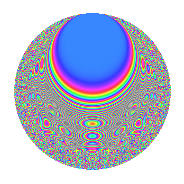# Properties

 Label 3234.2.cLevel 3234 Weight 2 Character orbit c Rep. character $$\chi_{3234}(197,\cdot)$$ Character field $$\Q$$ Dimension 164 Sturm bound 1344

# Related objects

## Defining parameters

 Level: $$N$$ $$=$$ $$3234 = 2 \cdot 3 \cdot 7^{2} \cdot 11$$ Weight: $$k$$ $$=$$ $$2$$ Character orbit: $$[\chi]$$ $$=$$ 3234.c (of order $$2$$ and degree $$1$$) Character conductor: $$\operatorname{cond}(\chi)$$ $$=$$ $$33$$ Character field: $$\Q$$ Sturm bound: $$1344$$

## Dimensions

The following table gives the dimensions of various subspaces of $$M_{2}(3234, [\chi])$$.

Total New Old
Modular forms 704 164 540
Cusp forms 640 164 476
Eisenstein series 64 0 64

## Trace form

 $$164q - 4q^{3} + 164q^{4} + 12q^{9} + O(q^{10})$$ $$164q - 4q^{3} + 164q^{4} + 12q^{9} - 4q^{12} + 8q^{15} + 164q^{16} - 140q^{25} - 4q^{27} - 8q^{31} - 8q^{33} - 8q^{34} + 12q^{36} - 16q^{37} + 8q^{45} - 4q^{48} - 16q^{55} + 40q^{58} + 8q^{60} + 164q^{64} + 20q^{66} - 32q^{67} + 48q^{69} + 92q^{75} - 40q^{78} + 20q^{81} + 16q^{82} + 8q^{93} + 64q^{97} + 16q^{99} + O(q^{100})$$

## Decomposition of $$S_{2}^{\mathrm{new}}(3234, [\chi])$$ into newform subspaces

The newforms in this space have not yet been added to the LMFDB.

## Decomposition of $$S_{2}^{\mathrm{old}}(3234, [\chi])$$ into lower level spaces

$$S_{2}^{\mathrm{old}}(3234, [\chi]) \cong$$ $$S_{2}^{\mathrm{new}}(33, [\chi])$$$$^{\oplus 6}$$$$\oplus$$$$S_{2}^{\mathrm{new}}(66, [\chi])$$$$^{\oplus 3}$$$$\oplus$$$$S_{2}^{\mathrm{new}}(231, [\chi])$$$$^{\oplus 4}$$$$\oplus$$$$S_{2}^{\mathrm{new}}(462, [\chi])$$$$^{\oplus 2}$$$$\oplus$$$$S_{2}^{\mathrm{new}}(1617, [\chi])$$$$^{\oplus 2}$$

## Hecke characteristic polynomials

There are no characteristic polynomials of Hecke operators in the database